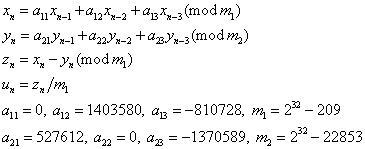ID 772987
Date 12/04/2020
Public

## MRG32k3a

This is a 32-bit combined multiple recursive generator with two components of order 3:MRG32k3a combined generator meets the requirements for modern RNGs, such as good multidimensional uniformity, or a long period. Optimization for various Intel® architectures makes it competitive with the other VS BRNGSs in terms of speed.

### Real Implementation (Single and Double Precision)

The output vector is the sequence of the floating-point values u0, u1, ...

### Integer Implementation

The output vector of 32-bit integers z0, z1, ...

### Stream Initialization by Function vslNewStream

MRG32k3a generates the stream and initializes it specifying the 32-bit input integer parameter seed. The stream state is the two triplets of 32-bit integers (x-1, x-2, ..., x-3 and y-1, y-2, ..., y-3), initialized in the following way:

1. Assume x-3 = seed modm1.

2. Assume the other values equal to 1, that is, x-2 = x-1 = y-3 = y-2 = y-1 = 1.

### Stream Initialization of Function vslNewStreamEx

MRG32k3a generates the stream and initializes it specifying the array n of 32-bit integer params[]:

1. If n = 0, assume x-3 = x-2 = x-1 = y-3 = y-2 = y-1 = 1.

2. If n = 1, assume x-3 = params mod m1, x-2 = x-1 = y-3 = y-2 = y-1 = 1.

3. If n = 2, assume x-3 = params mod m1, x-2 = params mod m1, x-1 = y-3 = y-2 = y-1 = 1.

4. If n = 3, assume x-3 = params mod m1, x-2 = params mod m1, x-1 = params mod m1, y-3 = y-2 = y-1 = 1.

If the values prove to be x-3 = x-2 = x-1 = 0, assume x-3 = 1.

5. If n = 4, assume x-3 = params mod m1,x-2 = params mod m1, x-1 = params mod m1, y-3 = params mod m2, y-2 = y-1 = 1.

If the values prove to be x-3 = x-2 = x-1 = 0, assume x-3 = 1.

6. If n = 5, assume x-3 = params mod m1, x-2 = params mod m1, x-1 = params mod m1, y-3 = params mod m2, y-2 = params mod m2, y-1 = 1.

If the values prove to be x-3 = x-2 = x-1 = 0, assume x-3 = 1.

7. If n ≥ 6, assume x-3 = params mod m1, x-2 = params mod m1, x-1 = params mod m1, y-3 = params mod m2, y-2 = params mod m2, y-1 = params mod m2.

If the values prove to be x-3 = x-2 = x-1 = 0, assume x-3 = 1.

If the values prove to be y-3 = y-2 = y-1 = 0, assume y-3 = 1.

### Subsequences Selection Methods

 vslSkipAheadStream Supported vslSkipAheadStreamEx Supported vslLeapfrogStream Not supported

### Generator Period### Lattice Structure

M8 = 0.68561,  M16 = 0.63940,  M32 = 0.63359.

### Empirical Testing Results Summary

Test Name
vsRngUniform
vdRngUniform
viRngUniform
viRngUniformBits
3D Spheres Test OK (10% errors) OK (10% errors) Not applicable OK (10% errors)
Birthday Spacing Test Not applicable Not applicable Not applicable OK (0% errors)
Bitstream Test Not applicable Not applicable Not applicable OK (20% errors)
Rank of 31x31 Binary Matrices Test Not applicable Not applicable Not applicable OK (20% errors)
Rank of 32x32 Binary Matrices Test Not applicable Not applicable Not applicable OK (10% errors)
Rank of 6x8 Binary Matrices Test Not applicable Not applicable Not applicable OK (0% errors)
Counts-the-1’s Test (stream of bits) Not applicable Not applicable Not applicable OK (20% errors)
Counts-the-1’s Test (stream of specific bytes) Not applicable Not applicable Not applicable OK (0% errors)
Craps Test OK (20% errors) OK (20% errors) OK (20% errors) OK (20% errors)
Parking Lot Test OK (10% errors) OK (10% errors) Not applicable OK (10% errors)
2D Self-Avoiding Random Walk Test OK (20% errors) OK (20% errors) Not applicable OK (20% errors)
NOTE:
1. The tabulated data is obtained using the one-level (threshold) testing technique. The OK result indicates FAIL < 50%. The run fails when p-value falls outside the interval [0.05, 0.95].

2. The stream tested is generated by calling the function vslNewStream with seed=7,777,777.